Question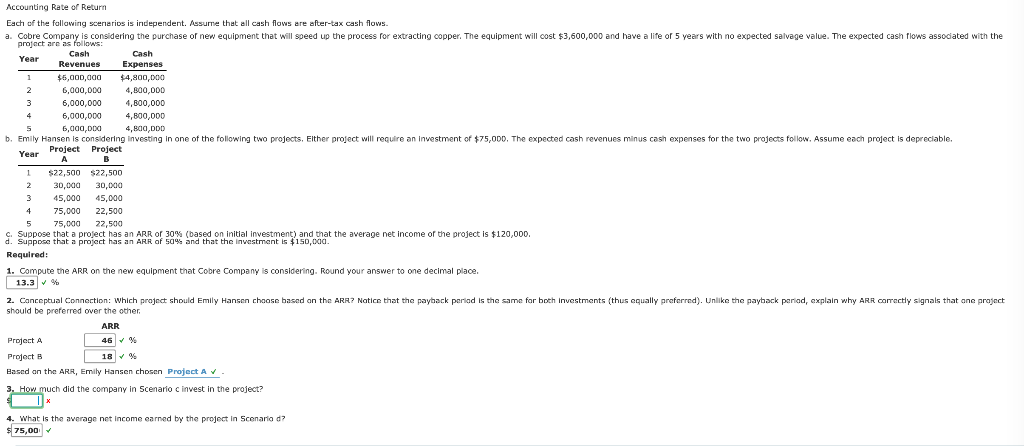Requirement 1 , 2 & 4 are already correctly answered as shown in image provided.

Requirement 3:

ARR is 30%

Average net income of project is \$120,000

Amount of investment is to be calculated

ARR = Average Net Income / Average Investment

here ARR is 30%

plotting values in formula,

30% = \$120,000 / Average investment

Average investment= \$ 120,000 / 30%

= \$ 400,000

therefore average investment is \$ 400,000 if ARR is 30% and net income is \$ 120,000

Company in scenario C invests \$ 400,000 in the project

#### Earn Coins

Coins can be redeemed for fabulous gifts.

Similar Homework Help Questions
• ### Accounting Rate of Retum Each of the following scenarios is independent. Assume that all cash flows are after-tax cash...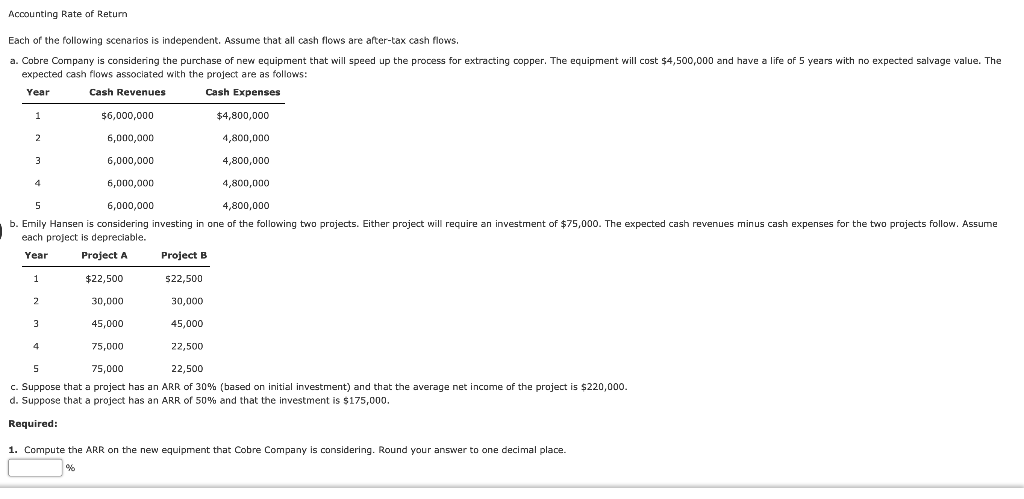Accounting Rate of Retum Each of the following scenarios is independent. Assume that all cash flows are after-tax cash flows. a. Cobre Company is considering the purchase of new equipment that will speed up the process for extracting copper. The equipment will cost \$4,500,000 and have a life of 5 years with no expected salvage value. The expected cash flows associated with the project are as follows: Cash Revenues Cash Expenses Year \$6,000,000 \$4,800,000 6,000,000 4,800,000 6,000,000 4,800,000 6,000,000 4,800,000...

• ### 12 Homework Each of the following scenarios is independent. Assume that all cash flows are after-tax...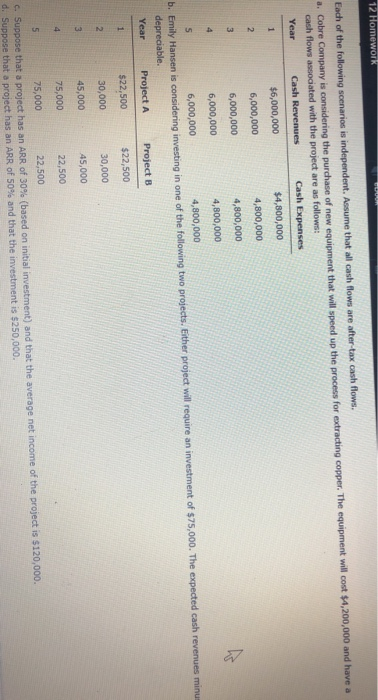12 Homework Each of the following scenarios is independent. Assume that all cash flows are after-tax cash flows. a. Cobre Company is considering the purchase of new equipment that will speed up the process for extracting copper. The equipment will cost \$4,200,000 and have a cash flows associated with the project are as follows: Year Cash Revenues Cash Expenses \$6,000,000 \$4,800,000 6,000,000 4,800,000 6,000,000 4,800,000 6,000,000 4,800,000 6,000,000 4,800,000 b. Emily Hansen is considering investing in one of the following...

• ### All scenarios are independent of all other scenarios. Assume that all cash flows are after-tax cash flows a. Kambry Da...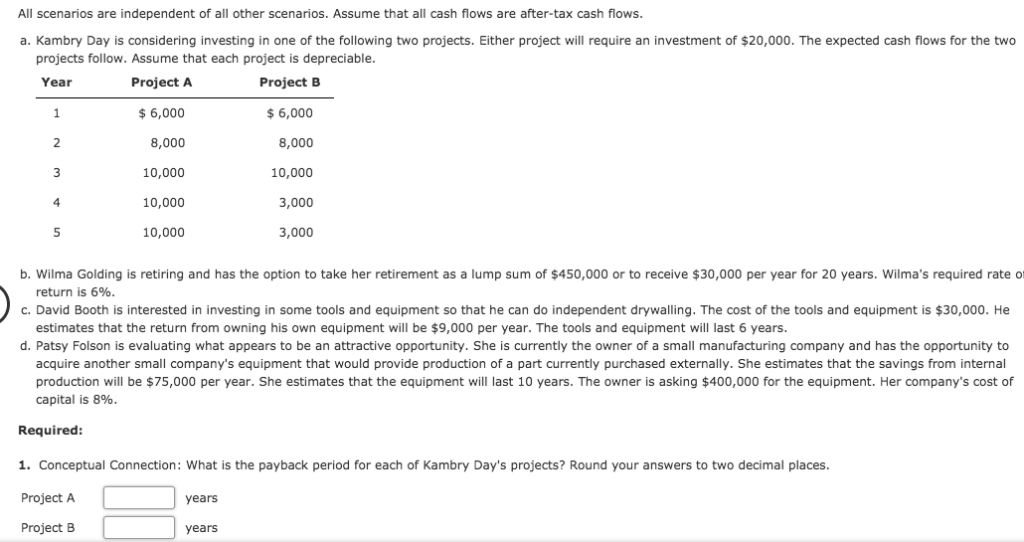All scenarios are independent of all other scenarios. Assume that all cash flows are after-tax cash flows a. Kambry Day is considering investing in one of the following two projects. Either project will require an investment of \$20,000. The expected cash flows for the two projects follow. Assume that each project is depreciable. Year Project A ProjectB 6,000 6,000 2 8,000 8,000 3 10,000 10,000 4 10,000 3,000 10,000 5 3,000 b. Wilma Golding is retiring and has the option...

• ### Payback, Accounting Rate of Return, Present Value, Net Present Value, Internal Rate of Return For discount...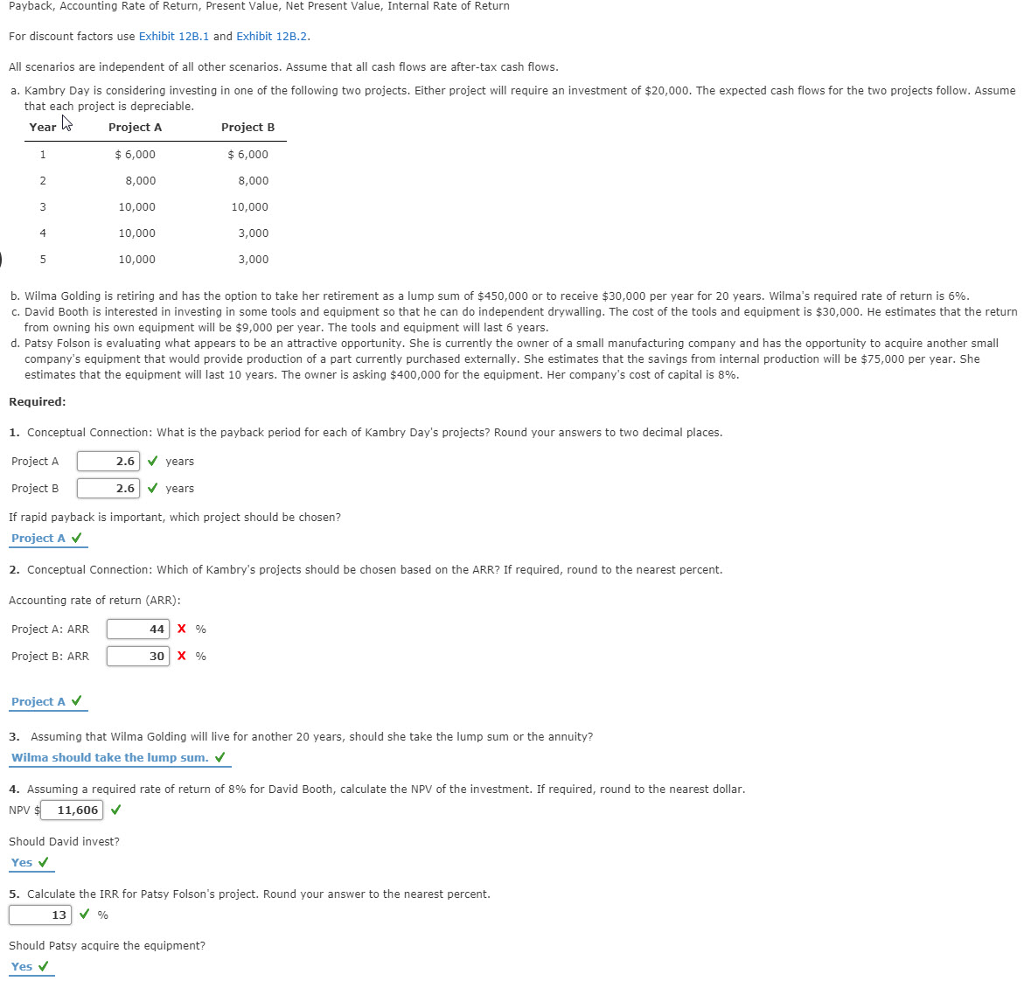Payback, Accounting Rate of Return, Present Value, Net Present Value, Internal Rate of Return For discount factors use Exhibit 12B.1 and Exhibit 12B.2 All scenarios are independent of all other scenarios. Assume that all cash flows are after-tax cash flows a. Kambry Day is considering investing in one of the following two projects. Either project will require an investment of \$20,000. The expected cash flows for the two projects follow. Assume that each project is depreciable. ProjectA 6,000 8,000 10,000...

• ### Your firm is considering two projects with the following cash flows: Cash flows from project B...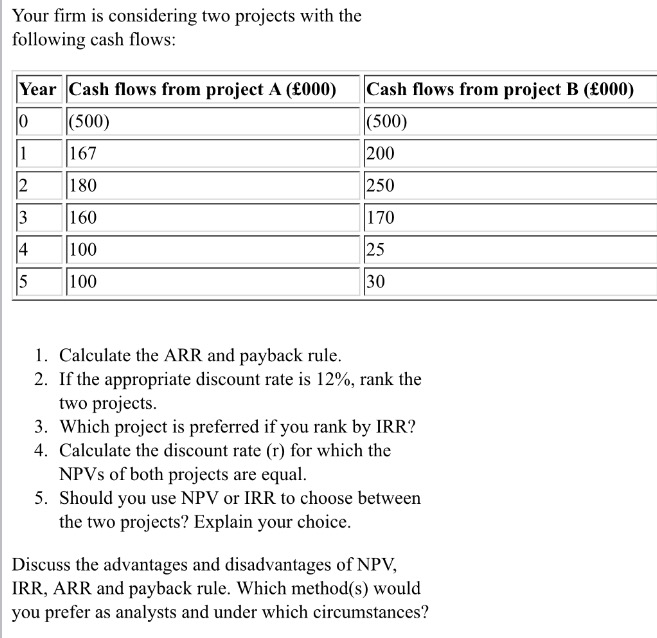Your firm is considering two projects with the following cash flows: Cash flows from project B (£000) (500) 200 250 170 25 30 Year Cash flows from project A (£000) 0(500) 167 180 160 100 100 4 1. Calculate the ARR and payback rule 2. If the appropriate discount rate is 12%, rank the two projects 3. Which project is preferred if you rank by IRR? 4. Calculate the discount rate (r) for which the NPVs of both projects are...

• ### Payback Period Each of the following scenarios is independent. Assume that all cash flows are after-tax...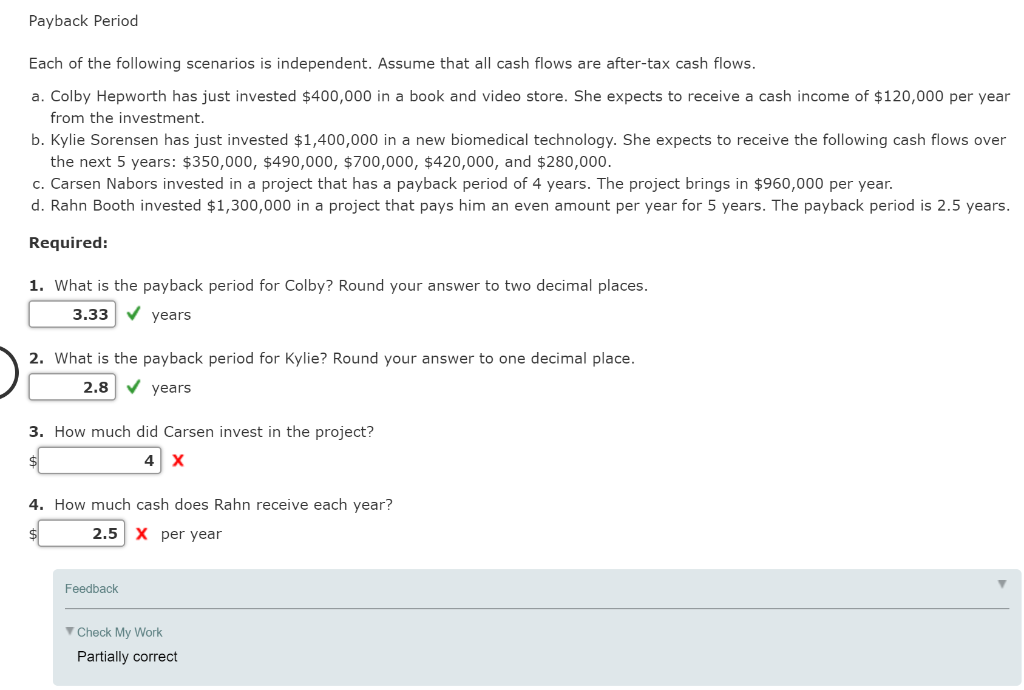Payback Period Each of the following scenarios is independent. Assume that all cash flows are after-tax cash flows. a. Colby Hepworth has just invested \$400,000 in a book and video store. She expects to receive a cash income of \$120,000 per year from the investment. b. Kylie Sorensen has just invested \$1,400,000 in a new biomedical technology. She expects to receive the following cash flows over the next 5 years: \$350,000, \$490,000, \$700,000, \$420,000, and \$280,000. c. Carsen Nabors invested...

• ### Each of the following scenarios is independent. Assume that all cash flows are after-tax cash flows....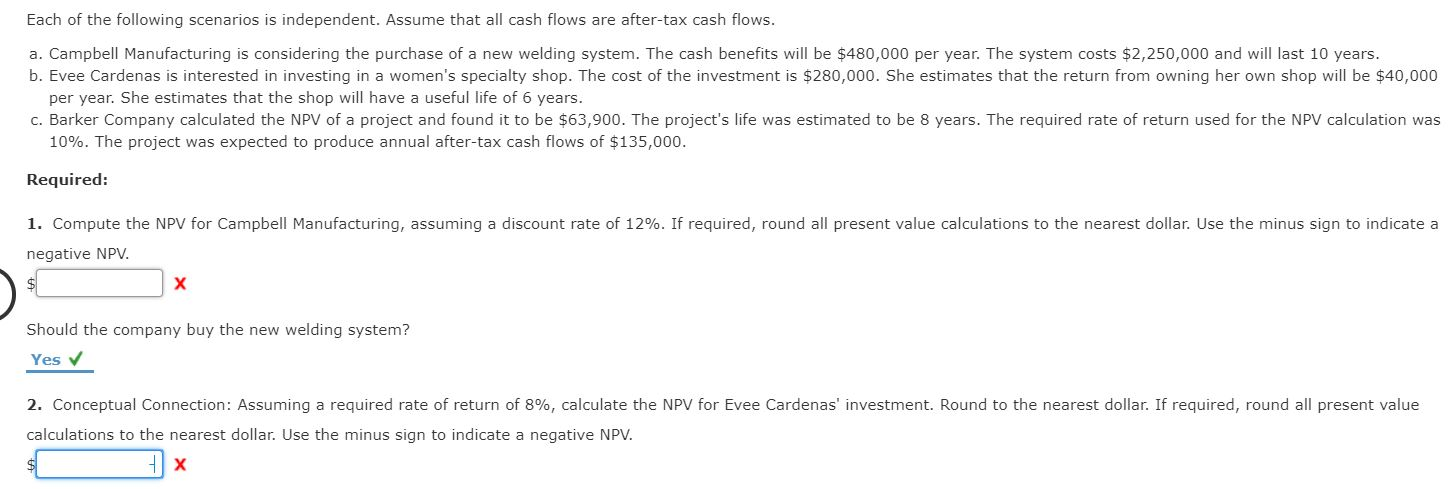Each of the following scenarios is independent. Assume that all cash flows are after-tax cash flows. a. Campbell Manufacturing is considering the purchase of a new welding system. The cash benefits will be \$480,000 per year. The system costs \$2,250,000 and will last 10 years. b. Evee Cardenas is interested in investing in a women's specialty shop. The cost of the investment is \$280,000. She estimates that the return from owning her own shop will be \$40,000 per year. She...

• ### Payback and ARR Each of the following scenarios is independent. All cash flows are after-tax cash...

Payback and ARR Each of the following scenarios is independent. All cash flows are after-tax cash flows. Required: 1. Brad Blaylock has purchased a tractor for \$92,500. He expects to receive a net cash flow of \$30,250 per year from the investment. What is the payback period for Jim? Round your answer to two decimal places. years 2. Bertha Lafferty invested \$385,000 in a laundromat. The facility has a 10-year life expectancy with no expected salvage value. The laundromat will...

• ### Payback and ARR Each of the following scenarios is independent. All cash flows are after-tax cash...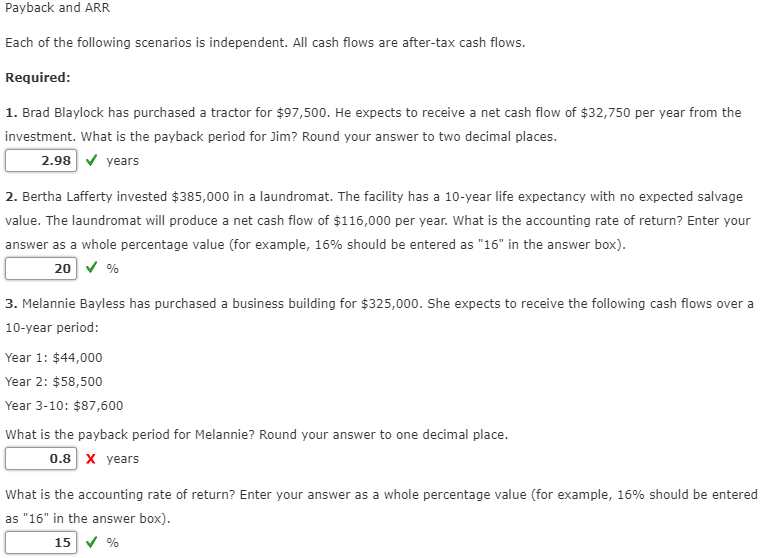Payback and ARR Each of the following scenarios is independent. All cash flows are after-tax cash flows. Required: 1. Brad Blaylock has purchased a tractor for \$97,500. He expects to receive a net cash flow of \$32,750 per year from the investment. What is the payback period for Jim? Round your answer to two decimal places. 2.98 years 2. Bertha Lafferty invested \$385,000 in a laundromat. The facility has a 10-year life expectancy with no expected salvage value. The laundromat...

• ### (Discounted payback period) Gio's Restaurants is considering a project with the following expected cash flows: Year...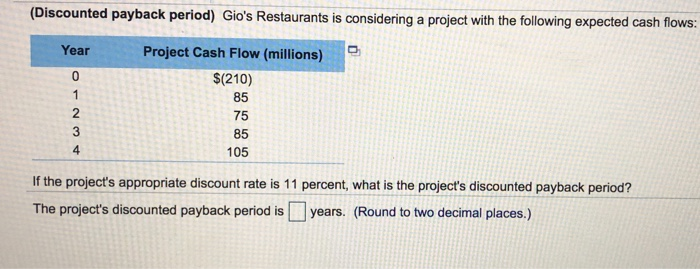(Discounted payback period) Gio's Restaurants is considering a project with the following expected cash flows: Year Project Cash Flow (millions) \$(210) AWNO If the project's appropriate discount rate is 11 percent, what is the project's discounted payback period? The project's discounted payback period is years. (Round to two decimal places.) (Mutually exclusive projects and NPV) You have been assigned the task of evaluating two mutually exclusive projects with the following projected cash flows: Project A Project B Year Cash Flow...